2017 A-level H1 Mathematics (8865) Paper 1 Suggested SolutionsAll solutions here are SUGGESTED. Mr. Teng will hold no liability for any errors. Comments are entirely personal opinions.

Numerical Answers (click the questions for workings/explanation)

Question 1:
Question 2:
Question 3:
Question 4:
Question 5:
Question 6:Question 7:Question 8:Question 9:;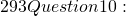latex 0.0336; \bar{y}=0.64, s^2 = 0.0400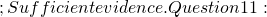latex \frac{48+x}{80+x}, \frac{32+x}{80+x}; x= 16; \frac{25}{32}; \frac{7}{16}; \frac{341}{8930}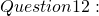latex 0.773; 0.0514; 0.866; 0.362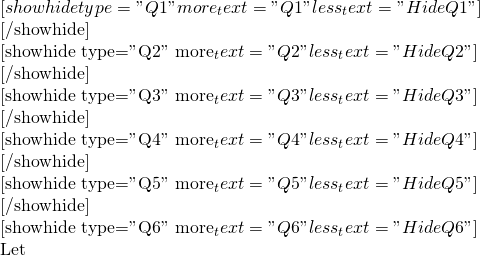latex X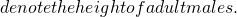latex X \sim \text{N} (\mu, \sigma^2) latex \text{P}( X \textless 1.6) = 0.2latex \text{P}( Z \textless \frac{1.6 – \mu}{\sigma}) = 0.2latex \frac{1.6 – \mu}{\sigma} = -0.8416212335 latex 1.6 = -0.8416212335 \sigma + \mulatex \text{P}( X \textgreater 1.75 ) = 0.3latex \text{P}( X \textless 1.75 ) = 0.7latex \text{P}( Z \textless \frac{1.75 – \mu}{\sigma} ) = 0.7latex \frac{1.75 – \mu}{\sigma} = 0.5244005101latex 1.75 = 0.5244005101 \sigma + \mulatex \mu = 1.69241, \sigma = 0.1098079154latex = 1.69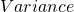latex = 0.0121latex Xlatex X \sim \text{B}(8, 0.7)latex \text{P}(X =5) = 0.254latex Ylatex Y \sim \text{B}(8, 0.3)latex \text{P}(Y \ge 4)latex = 1 – \text{P}(Y le 3)latex = 0.19410435 \approx 0.194latex Wlatex W \sim \text{B} (6, 0.19410435)latex \text{P} ( W \le 2)latex = 0.9082304639 \approx 0.908latex {{6}\choose{3}} \times 3! \times {{8}\choose{3}} \times 3! = 40320latex \bigg[ {{5}\choose{1}} \times \frac{3!}{2!} + {{5}\choose{2}} \times 3! \bigg] \times {{7}\choose{1}} \times \frac{3!}{2!} = 1575latex = \frac{1575}{6^3 \times 8^3} = 0.0142latex \text{P}(\text{has~}2\text{~as~its~first~character}) = \frac{1}{6}latex \text{P}(\text{has~H~as~its~sixth~character}) = \frac{1}{8}latex \text{P}(\text{has~}2\text{~as~its~first~character~and~has~H~as~its~sixth~character}) = \frac{1}{6 \times 8} = \frac{1}{48}latex = \frac{1}{6} + \frac{1}{8} – 2(\frac{1}{48}) = \frac{1}{4}latex \text{r}=0.978latex a=0.182, b=2.56latex y = 0.1821185456 x + 2.564419724latex x = 2, y = 0.1821185456 (2) + 2.564419724latex \Rightarrow y = 2.9287293.

(v)
Firstly, the linear model is not appropriate here. Since it suggests as the number of years increases, the weekly earnings will increase proportionately, which is not realistic.

Secondly,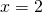is out of data range and this is extrapolation, which is a bad practice since our trend might not continue out of data range.
[/showhide]

Relevant materials

MF26

•Leah

•KS Teng

yes, sorry was having a H2 math class for J2 just now.

•Leah

it’s ok!! Thank u for doing this 🙂

Not readable? Change text.